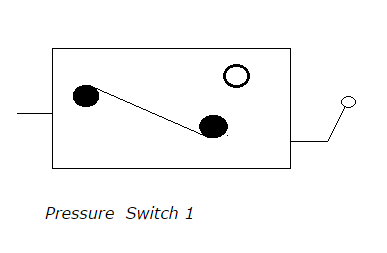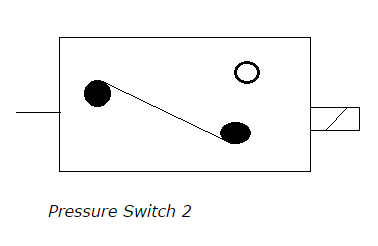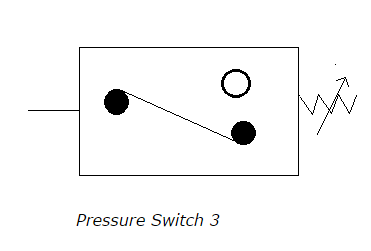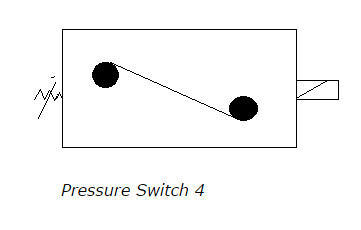# Accumulators and Intensifiers - MCQs with Answers

## Accumulators and Intensifiers – MCQs with Answers

1. Which of the following statements are true for accumulator used in hydraulic systems?

1. accumulator stores fluid with pressure
2. accumulator stores fluid without any pressure
3. accumulator stores compressible liquid
4. spring is used as an external source to keep the fluid under hydraulic pressure

a. 1, 3 and 4
b. 2 and 3
c. 1 and 4
d. 2, 3 and 4

2. Which of the following is a gas charged accumulator?

c. weighted accumulator
d. all the above

3. How is pressure of fluid under piston calculated in a weighted accumulator?

a. pressure of fluid = (weight added / piston area)
b. pressure of fluid = (piston area / weight added)
c. pressure of fluid = (weight added / piston force)
d. pressure of fluid = (piston force / weight added)

4. Which of the following gas is used in gas charged accumulator?

a. oxygen
b. nitrogen
c. carbon dioxide
d. all the above

5. The relation for rapid change in pressure and volume adiabatically is given as

a. p0 v0 = p1 v1 = p2 v2
b. p0 v0 = p1 v1n = p2 v2n
c. p0 v0n = p1 v1n = p2 v2n
d. none of the above

ANSWER: c. p0 v0n = p1 v1n = p2 v2n

6. Accumulator used in gas charged accumulator is

a. hydraulic
b. pneumatic
c. hydropneumatic
d. none of the above

7. What is the function of pressure switch?

a. pressure switch is used to start a motor
b. pressure switch is used to stop a motor
c. pressure switch is used to deenergize a solenoid
d. all the above

8. Which symbol represents a pressure switch?

a.b.c.d.9. Intensifier used in pneumatic systems has output pressure

a. less than input pressure
b. more than input pressure
c. same as input pressure
d. none of the above

ANSWER: b. more than input pressure

10. What is the function of unloading relief valve and can it be used as an accessory for accumulators?

a. unloading relief valve is used to charge the accumulator by a pump when accumulator pressure falls below the set value and it can be used as an accessory.

b. unloading relief valve is used to charge the accumulator by a pump when accumulator pressure falls below the set value but is not used as an accessory

c. unloading relief valve is used to charge the accumulator by a pump when accumulator pressure rises above the set value but is not used as an accessory

d. unloading relief valve is used to charge the accumulator by a pump when accumulator pressure rises above the set value and is used as an accessory